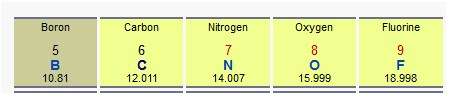Looking for revision notes that are specific to the exam board you are studying? If so, click the links below to view our condensed, easy-to-understand revision notes for each exam board, practice exam question booklets, mindmap visual aids, interactive quizzes, PowerPoint presentations and a library of past papers directly from the exam boards.

Formulae Equations and Amount of Substance

Key Facts & Summary:

• Formulae are used for writing the atoms forming a molecule.
• The molecular formula, simply shows the type of atom in a molecule and the number of times they are present in the entire molecule.
• The empirical formula is just showing the ratio between the atoms, thus it express the simplest positive integer ratio of atoms present in a compound.
• An empirical formula makes no mention of the arrangement or number of atoms.
• The atomic mass is the mass of an atom given in unified atomic mass units (u, or Da). One unified atomic mass unit is defined as ​1⁄12 of the mass of a single carbon-12 atom.
• The mole is the unit of measurement for amount of substance in the International System of Units (SI). Each mole contains exactly 6.02214076×1023 number of constitutive particles.
• The molar mass M for atoms it is given by the Standard atomic weight of the element multiplied by the molar mass constant
• The molecular weight or molecular mass is the sum of the standard atomic weight of the atoms which form the compound multiplied by the molar mass constant.

Empirical and molecular formulae

Formulae are used for writing the atoms forming a molecule. There are two main way how to write it.

The molecular formula, simply shows the type of atom in a molecule and the number of times they are present in the entire molecule.

The empirical formula is just showing the ratio between the atoms, thus it express the simplest positive integer ratio of atoms present in a compound. It is possible that empirical and molecular formulae are the same from the same compound, especially for small molecules, like water.

It is also possible for different types of compounds to have equal empirical formulas.

Let’s make an example:

Ethylene is composed of 2 Carbon atom and 4 Hydrogen. the molecular formula would just count all of them and it will  be C2H4.  The empirical formula will just express the minimum ration between the atoms so it will be CH2. It is like saying that for each Carbon atom there are 2 Hydrogens.An empirical formula makes no mention of the arrangement or number of atoms. For example, the molecule C6H12O6 could be glucose, fructose, galactose, or another simple sugar.

Formulas are determined experimentally measuring them via Elemental analysis. It is a process where a sample of some material is analyzed for its elemental and sometimes isotopic composition. Elemental analysis can determine what elements are present (qualitative) or determining the amount of each element is present (quantitative).

Atomic mass

The atomic mass is the mass of an atom given in unified atomic mass units (u, or Da). One unified atomic mass unit is defined as ​1⁄12 of the mass of a single carbon-12 atom. For atoms, the protons and neutrons of the nucleus account for almost all of the mass, and the atomic mass measured in u has nearly the same value as the mass number.

The International Union of Pure and Applied Chemistry (IUPAC) defined the standard atomic weight (Ar, standard, a relative atomic mass) for each element reflecting the variance of natural isotopes. These values are reported for each element in the periodic table.The mole

The mole (mol) is the unit of measurement for amount of substance in the International System of Units (SI). Each mole contains exactly 6.02214076×1023 number of constitutive particles. 6.02214076×1023 is called Avogadro’s constant (Ag).

To understand better, let’s make a basic example.

You know that at the supermarket they sell eggs in boxes of 6 pieces. Every box contains 6 eggs. So if you want to ask your mother to buy 12 eggs, you can either ask for 12 eggs or for 2 boxes of eggs. The mole is the “box” we use for atoms or molecules.

If we have a mole of Na+ in a glass of water it means that we have 6.02214076×1023 ions of sodium in the glass.

If we have 2 moles of Na+ in a glass of water it means that we have 2 * Ag ions of sodium in the glass. And so on.

This concept is very useful for calculating the amount of substances macroscopically.

Each substance has a molar mass M.

For atoms it is given by the Standard atomic weight of the element multiplied by the molar mass constant, u = 1 × 10−3 kg/mol = 1 g/mol. This ensures that the calculation is dimensionally correct since standard relative atomic masses are dimensionless quantities (i.e., pure numbers). So the Standard atomic weight number is the same of the molar mass for elements, just the units is changing.

M(H) = 1.007 97(7) × 1 g/mol = 1.007 97(7) g/mol

Another way how to define the molar mass is: the mass divided by the amount of substance in moles. In other words it is the mass in grams of each mole. The base SI unit for molar mass is kg/mol. However, for historical reasons, molar masses are almost always expressed in g/mol.

The molecular weight or molecular mass is the sum of the standard atomic weight of the atoms which form the compound multiplied by the molar mass constant.

examples:

M(NaCl) = [22.989 769 28(2) + 35.453(2)] × 1 g/mol = 58.443(2) g/mol

M(H2O) ≈ 18.015 g/mol.